## 14.4 深度RNNn_neurons = 100
n_layers = 3

basic_cell = tf.contrib.rnn.BasicRNNCell(num_units=n_neurons)
multi_layer_cell = tf.contrib.rnn.MultiRNNCell([basic_cell] * n_layers)
outputs, states = tf.nn.dynamic_rnn(multi_layer_cell, X, dtype=tf.float32)


### 14.4.2 应用Dropout

keep_prob = 0.5

cell = tf.contrib.rnn.BasicRNNCell(num_units=n_neurons)
cell_drop = tf.contrib.rnn.DropoutWrapper(cell, input_keep_prob=keep_prob)
multi_layer_cell = tf.contrib.rnn.MultiRNNCell([cell_drop] * n_layers)
rnn_outputs, states = tf.nn.dynamic_rnn(multi_layer_cell, X, dtype=tf.float32)


## 14.5 LSTM Cell

lstm_cell = tf.contrib.rnn.BasicLSTMCell(num_units=n_neurons)


LSTM cell要管理两个状态向量，为了性能原因它们默认是分开的。可以在创建BasicLSTMCell的时候设置state_is_tuple=False来改变这一行为。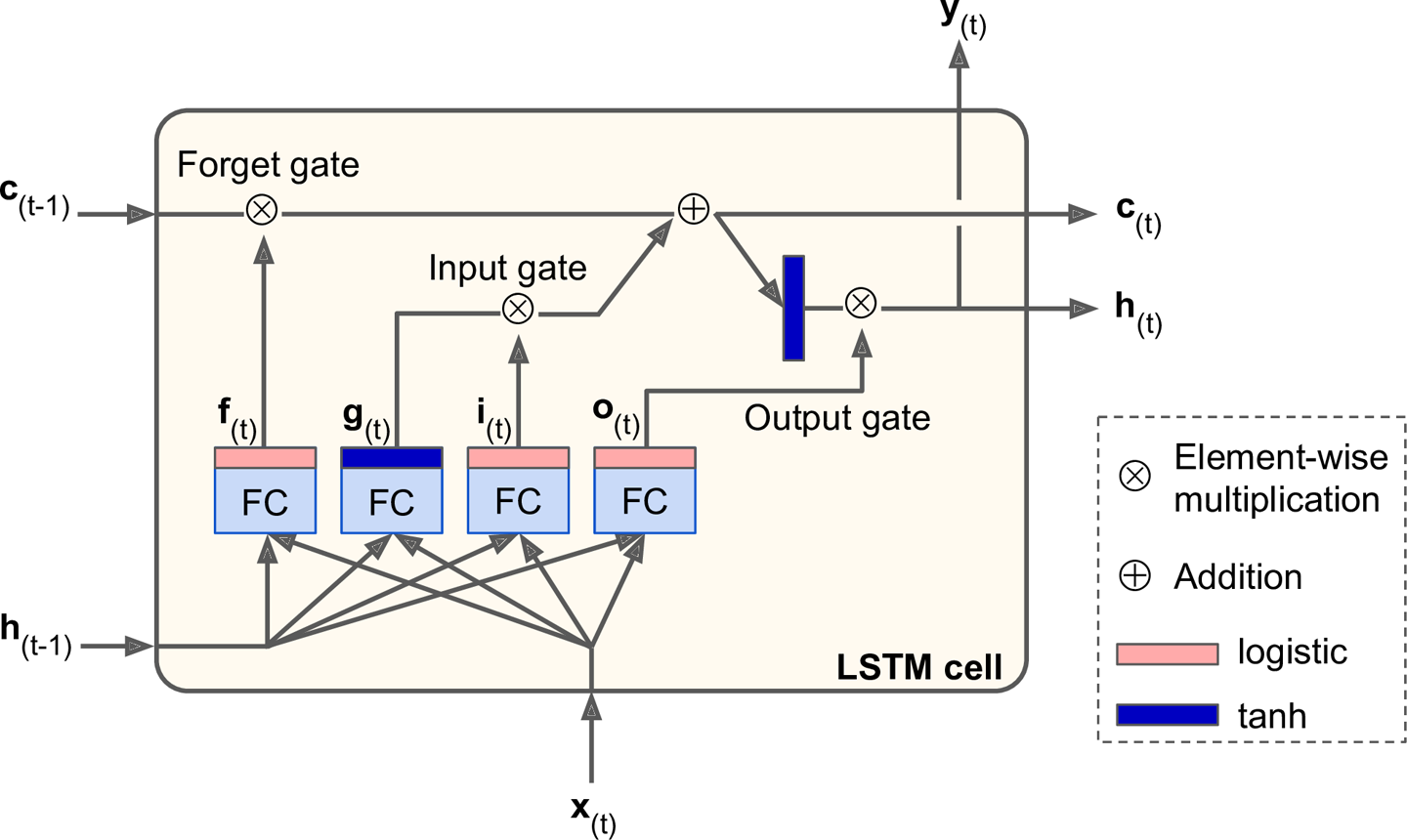• 最重要的一层是输出$\textbf{g}_{(t)}$的那个。它拥有类似基本cell的分析当前时刻输入$\textbf{x}_{(t)}$和前一时刻短期记忆$\textbf{h}_{(t-1)}$的角色。对于基本的cell，$\textbf{y}_{(t)}$和$\textbf{h}_{(t)}$会直接输出。不过LSTM cell的这一层不会直接输出，还会部分地保存在长期记忆中。
• 另外的三层是门控制器（gate controllers）。他们使用logistic激活函数，输出的范围是0到1。其输出用于按元素点乘运算。所以如果输出0，门被关闭；如果输出1，门被打开。明确来讲：
• 遗忘门（forget gate，由$\textbf{f}_{(t)}$控制）决定哪些长期记忆应该被遗忘。
• 输入门（input gate，由$\textbf{i}_{(t)}$控制）决定$\textbf{g}_{(t)}$的哪些内容应该被添加到长期记忆。
• 输出门（output gate，由$\textbf{o}_{(t)}$控制）决定哪些长期记忆应该被读取和输出。

LSTM一个实例输出的计算公式：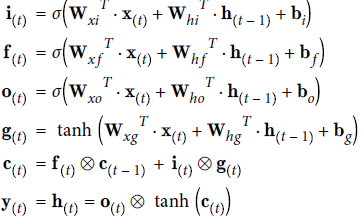• $W_{xi},W_{xf},W_{xo},W_{xg}$是4个全连接层关于输入向量$\textbf{x}_{(t)}$的权重矩阵。
• $W_{hi},W_{hf},W_{ho},W_{hg}$是4个全连接层关于短期记忆$\textbf{h}_{(t-1)}$的权重矩阵。
• $b_{i},b_{f},b_{o},b_{g}$是4个全连接层的偏置项。TensorFlow会将$b_{f}$初始化为全是1的矩阵，而不是全是0，这会使得训练初期没有东西被遗忘。

### 14.5.1 Peephole Connections

lstm_cell = tf.contrib.rnn.LSTMCell(num_units=n_neurons, use_peepholes=True)


## 14.6 GRU Cell

Gated Recurrent Unit (GRU) cell在2014年的一篇论文中提出， 该论文同时提出了我们先前提到的Encoder–Decoder神经网络。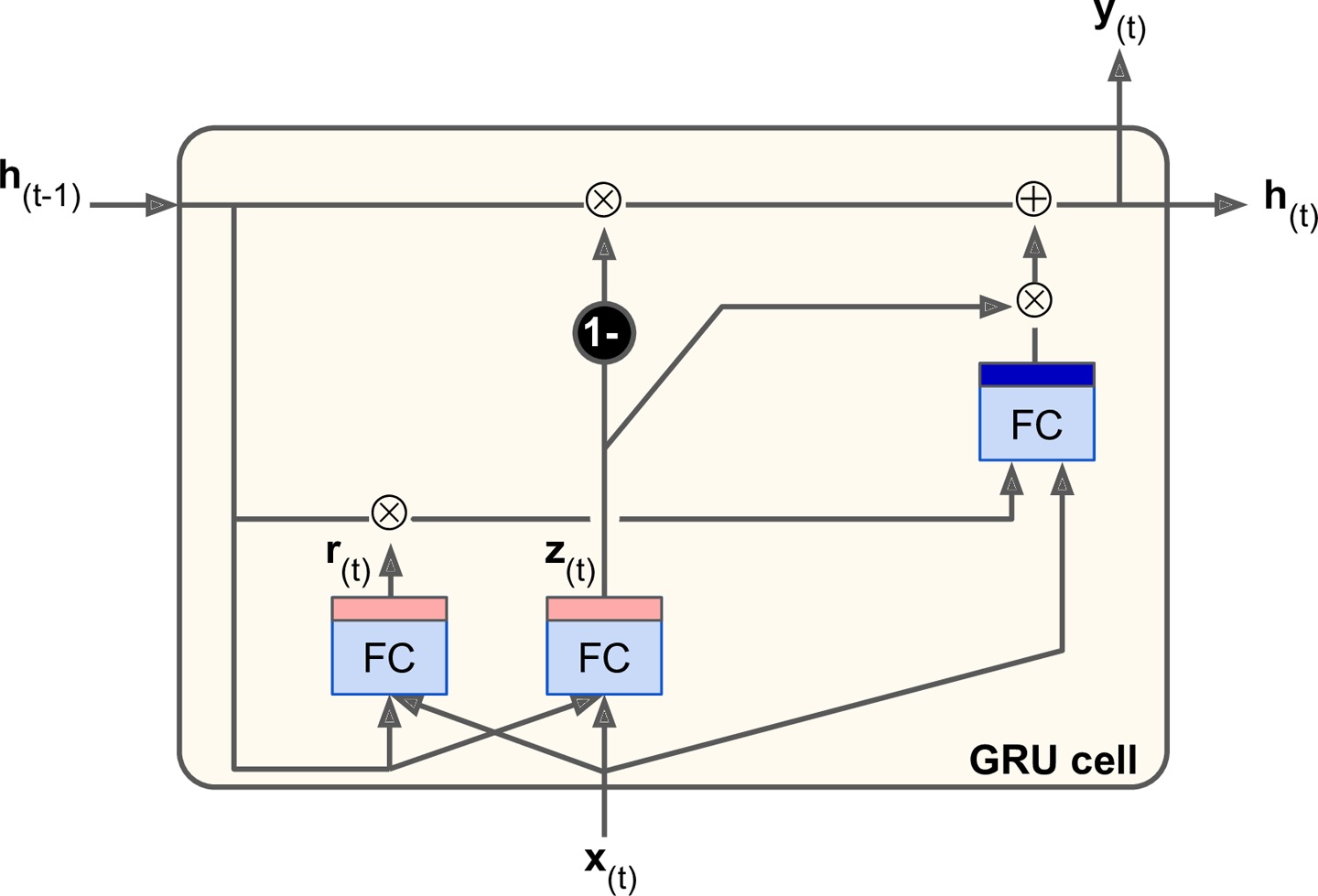GRU cell是LSTM cell的简化版，但是表现的同样好（2015年的论文LSTM: A Search Space Odyssey表明，所有LSTM变种的表现大致相同）。主要简化的部分如下：

• 两个状态向量被合并成了单独的$\textbf{h}_{(t)}$。
• 一个门控制器（$\textbf{z}_{(t)}$）同时控制遗忘门和输入门。如果门控制器输出1，输入门被打开同时遗忘门被关闭。如果控制器输出0，输入门被关闭同时遗忘门被打开。换句话说，如果一个记忆需要被存储，那么该位置原先的记忆会被清除。
• 不再使用输出门，整个状态矩阵都会输出。不会，有一个新的门控制器（$\textbf{h}_{(r)}$），来控制先前的哪些记忆需要传递给主层。

GRU一个实例输出的计算公式：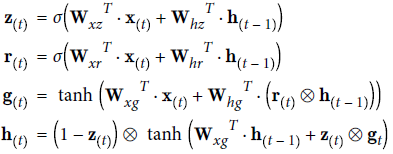gru_cell = tf.contrib.rnn.GRUCell(num_units=n_neurons)


LSTM和GRU cells是近些年RNNs取得成功的重要原因，尤其是在自然语言处理领域。

## 14.7 自然语言处理

### 14.7.1 Word Embeddings

vocabulary_size = 50000
embedding_size = 150
embeddings = tf.Variable(tf.random_uniform([vocabulary_size, embedding_size], -1.0, 1.0))


train_inputs = tf.placeholder(tf.int32, shape=[None]) # from ids...
embed = tf.nn.embedding_lookup(embeddings, train_inputs) # ...to embeddings


### 14.7.2 基于Encoder–Decoder神经网络的机器翻译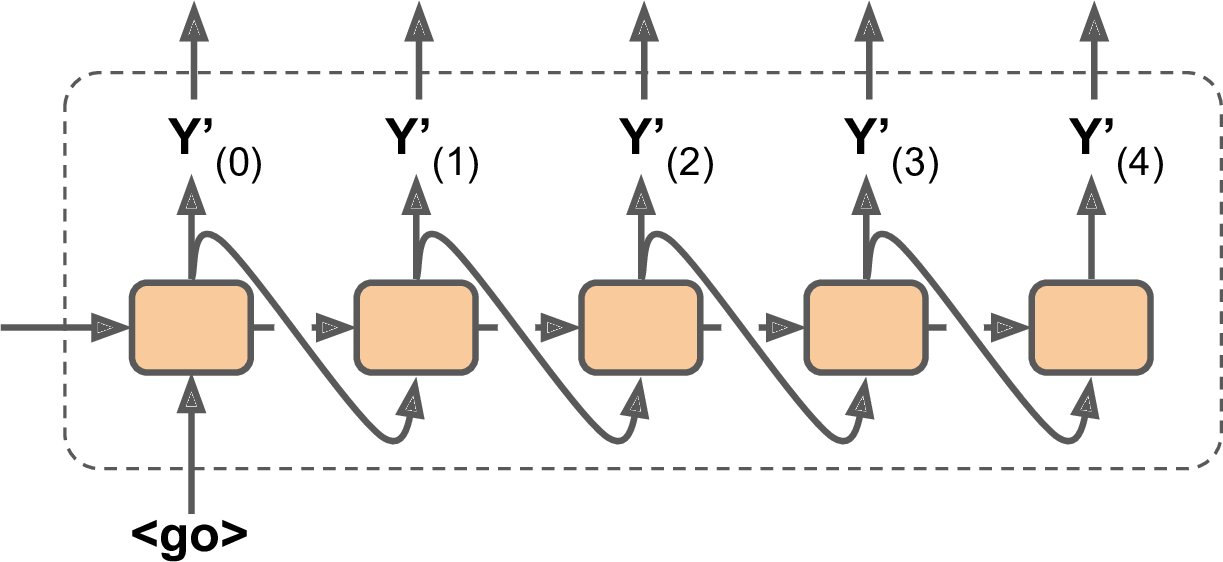• 首先，我们假设了所有的输入序列（包括encoder和decoder）都是定长的。但是很明显，句子的长度是不定的。这有多种处理方式——比如，static_rnn()和dynamic_rnn()函数使用sequence_length参数来描述句子的长度。不过，教程中使用了另一个方案（可能是由于性能原因）：将一个句子切割成不同的组，每组长度相同（比如，一个矩阵的1-6个单词分为一组，7-12个单词分为另一组，等等）。较短的组使用特殊标记（比如“<pad>”）进行填充。例如“I drink milk”转换成“<pad> <pad> <pad> milk drink I”，然后翻译为“Je bois du lait <eos> <pad>”。当然，我们希望忽略EOS之后的内容。教程中的实现方式是使用一个target_weights向量，。比如对于目标语句“Je bois du lait <eos> <pad>”，这一向量是[1.0, 1.0, 1.0, 1.0, 1.0, 0.0]（进行填充的位置就是0.0）。
• 其次，由于词表很大，输出每个词可能的概率去计算交叉熵是很慢的。一个解决方案是decoder输出一个小得多的向量，比如1000维，然后使用抽样技术估计损失。这一Sampled Softmax技术在2015年被提出。在TensorFlow中可以使用sampled_softmax_loss()函数。
• 其次，教程中的实现使用了attention机制（attention mechanism）。RNN的attention机制超出了本书的范围，不过可以参考machine translationmachine readingimage captions
• 最后，教程中的实现使用了tf.nn.legacy_seq2seq模块，这使得创建多种Encoder–Decoder模型变得简单。比如，embedding_rnn_seq2seq()创建的Encoder–Decoder模型自动进行word embeddings。

posted on 2018-05-26 18:02  royhoo  阅读(4297)  评论(0编辑  收藏  举报